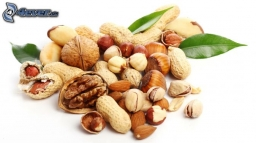# Nutballs

The dough for nutballs contains, among other things, two basic raw materials: flour and nuts in a ratio of 2:1. How much is flour, and how many nuts are needed for 1 kg of dough if "other" is 100g?

m =  600 g
o =  300 g

### Step-by-step explanation:Did you find an error or inaccuracy? Feel free to write us. Thank you!

Tips for related online calculators
Check out our ratio calculator.
Do you have a linear equation or system of equations and looking for its solution? Or do you have a quadratic equation?
Do you want to convert mass units?